Next: Continuous Spectra Up: Fundamental Concepts Previous: Compatible Observables

# Uncertainty Relation

We have seen that if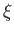and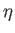are two noncommuting observables then a determination of the value ofleaves the value ofuncertain, and vice versa. It is possible to quantify this uncertainty. For a general observable, we can define an Hermitian operator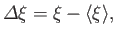(1.71)

where the expectation value is taken over the particular physical state under consideration. It is obvious that the expectation value of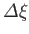is zero. The expectation value of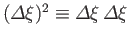is termed the variance of, and is, in general, non-zero. In fact, it is easily demonstrated that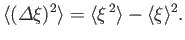(1.72)

The variance ofis a measure of the uncertainty in the value offor the particular state in question (i.e., it is a measure of the width of the distribution of likely values ofabout the expectation value). If the variance is zero then there is no uncertainty, and a measurement ofis bound to give the expectation value,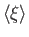.

Consider the Schwarz inequality,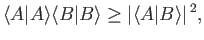(1.73)

which is analogous to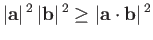(1.74)

in Euclidian space. This inequality can be proved by noting that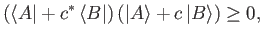(1.75)

where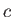is any complex number. Iftakes the special value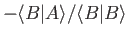then the previous inequality reduces to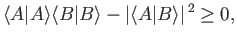(1.76)

which is equivalent to the Schwarz inequality.

Let us substitute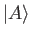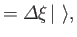(1.77)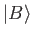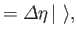(1.78)

into the Schwarz inequality, where the blank ket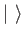stands for any general ket. We find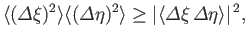(1.79)

where use has been made of the fact thatand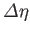are Hermitian operators. Note that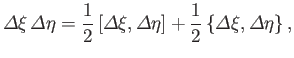(1.80)

where the commutator,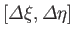, and the anti-commutator,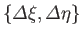, are defined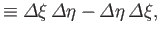(1.81)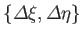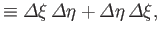(1.82)

respectively. The commutator is clearly anti-Hermitian,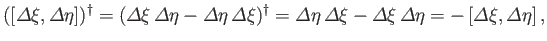(1.83)

whereas the anti-commutator is obviously Hermitian. Now, it is easily demonstrated that the expectation value of an Hermitian operator is a real number, whereas the expectation value of an anti-Hermitian operator is an imaginary number. (See Exercise 11.) It follows that the right-hand side of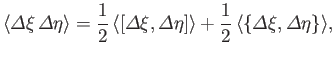(1.84)

consists of the sum of an imaginary and a real number. Taking the modulus squared of both sides gives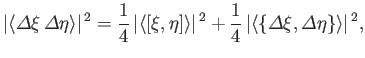(1.85)

where use has been made of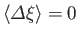, et cetera. The final term on the right-hand side of the previous expression is positive definite, so we can write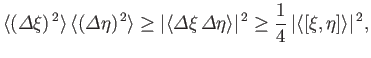(1.86)

where use has been made of Equation (1.79). The previous expression is termed the uncertainty relation. According to this relation, an exact knowledge of the value of(i.e.,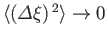) implies no knowledge whatsoever of the value of(i.e.,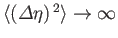), and vice versa. The one exception to this rule is whenandcommute, in which case exact knowledge ofdoes not necessarily imply no knowledge of.Next: Continuous Spectra Up: Fundamental Concepts Previous: Compatible Observables
Richard Fitzpatrick 2016-01-22